# Queues of Cubes

Here is a nice problem tweeted by the Republic of Mathematics:

Free of the constraints of Twitter’s 140 character limit, let’s explain this problem in a little more detail.

The first part of the tweet claims that the mean of any three consecutive cubes is an integer. When you think about problems like this, it is always useful to look at a few actual examples to get a feel for what is happening, so let’s check a few arbitrary cases, to satisfy ourselves that the statement is probably true:

• (1³ + 2³ + 3³) / 3 = (1 + 8 + 27) / 3 = 36 / 3 = 12
• (9³ + 10³ + 11³) / 3 = (729 + 1000 + 1331) / 3 = 3060 / 3 = 1020
• (117³ + 118³ + 119³) / 3 = (1601613 + 1643032 + 1685159) / 3 = 4929804 / 3 = 1643268

Everything seems in order here. Three examples don’t make a proof, of course, but we can at least say that this fact is not obviously false.

The second part of the tweet asks whether this fact is true in a more general sense. Specifically, it asks whether the mean of consecutive pth powers is always an integer, where p could be any positive odd number.

Once again, picking a few examples does not turn up any counter-examples. Both prime and composite odd numbers seem to work fine. Here are a few cases from the many that I checked:

• (15 + 25 + 35 + 45 + 55) / 5 = (1 + 32 + 243 + 1024 + 3125) / 5 = 4425 / 5 = 885
• (1315 + 1415 + … + 2715) / 15 = 6598745288552260908000 / 15 = 439916352570150727200
• (2327 + 2427 + … + 4927) / 27 = 367153658307214890160236378837616908060361848

OK, so our working hypothesis is that the statement is true. How can we prove it?

Well, let’s start by writing the statement in an algebraic form. We want to prove that: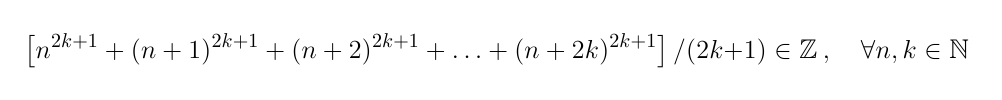Here, we are using 2k + 1 to represent our arbitrary odd number p, where k could be any positive integer.* This is more practical than working with odd numbers directly. The first power in our sum is n2k + 1 and we are adding 2k + 1 consecutive powers in total, up to (n + 2k)2k + 1 , then dividing by 2k + 1 to find the mean. The question is about whether the result always lies in the set of integers, Z.

It will be handy to write the statement a little more concisely: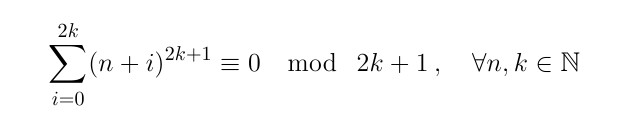Now, as well as rolling the sum up into the more efficient Sigma notation, rather than stating that the mean of the powers should be an integer, we are stating that their sum should be congruent to 0 modulo 2k + 1 (i.e. there should be no remainder when the sum is divided by 2+ 1). This is equivalent, but more mathematically convenient.

To prove this statement, we are going to do a bit of mathematical induction with respect to the base of the first power: n. First we will show that the statement is true (for all k) when n = 0; then we will show that if the statement is true when n is equal to some arbitrary value N, then it is also true when n_=_N_+_1. By induction, this will prove the statement for every positive integer n.

First then, we will set n = 0. In other words,  we will only consider sums of consecutive powers that begin with a power of zero. In fact, since any power of zero is equal to zero, we can actually ignore the first term and start our sum from the power of 1, so this is what we want to prove: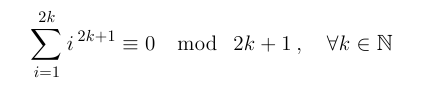Our next step is a bit of a trick. The summation on the left hand side has 2k terms. We can add them up in any order we like, so let’s rearrange them into pairs; the first with the last, the second with the second-to-last, and so on… This gives us a new sum with k terms: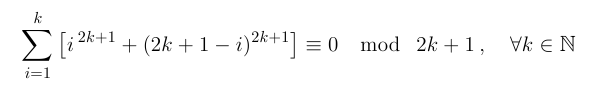Now we are going to make use of the fact that we are working modulo 2k + 1. This allows us to throw away any multiples of 2k + 1 that sneak into our sum. If we expand the internal brackets as a binomial (with 2k + 1 and –i as the two terms), all but one term has 2k + 1 as a factor, so this considerably simplifies the expression: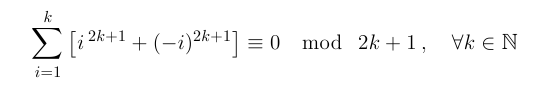Raising a negative number to an odd power gives you the negative of the positive power, so this moves us rapidly on to: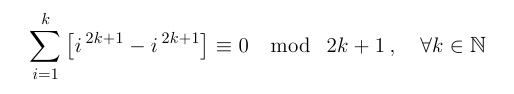All we have done here is rewritten the statement that we are trying to prove (for n = 0), but now it has more-or-less proven itself, since the terms of our sum are clearly all equal to zero! So we have shown that the mean of the first consecutive pth powers (starting from 0p) is always an integer, provided that p is odd.

We have proved the original statement for n = 0. Now we have to perform the second part of the induction; proving that if the statement is true for n = N, then it is true for n = N + 1.

First, we will write the statement for n = N and assume that it is true: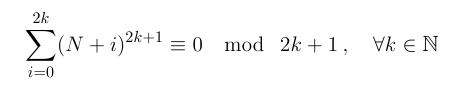To achieve our goal, we will show that adding the next term to this sum and removing the first – thus transforming the statement for n = N into the statement for n = N + 1 – has no effect on the congruence modulo 2k + 1. This will be true if the difference of these two terms is equal to zero modulo 2k + 1, so we need to prove that: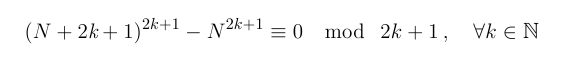A binomial expansion will help us out once again here, because expanding that first bracket as a binomial – with N and 2k + 1 as the two terms – results in only one term, N2k + 1, that is not clearly a multiple of 2k + 1. All those multiples of 2k + 1 can be disregarded when working congruent to 2k + 1, so this statement becomes.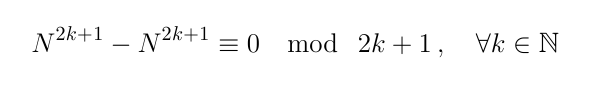This is clearly true, so we can go ahead and add (N + 2k + 1)2k + 1 – N2k + 1  to our summation without invalidating it, giving us:As intended, the negative N2k + 1 cancels the first term of the sum, while the (N + 2k + 1)2k + 1 fits in nicely as the next term. With a nifty bit of reindexing (shifting i to become i + 1) the left hand side can all be wrapped up into a single sum, like this: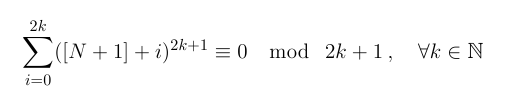But this is the same statement that we assumed to be true earlier, with N replaced by N + 1, thus completing the second part of the induction. We have shown that if the statement is true for n = N then it is true for n = N + 1, and since we already know that it is true for n = 0, by induction, it must be true for all non-negative integers n.

So the statement proposed by the Republic of Maths is true. The mean of any p consecutive pth powers is indeed always an integer, where p can be any positive odd number.

Nice number fact.

* The problem is trivial when k = 0.

Thomas Oléron Evans, 2015

## 3 thoughts on “Queues of Cubes”

1.David Morales

Hi! This post about queues of cubes, helped me to find a relationship between the average of the cube of three consecutive numbers and the OEIS sequence A54602: the product and sum of three consecutive numbers is also the average of the cubes of those three consecutive numbers, so your post has been linked there with reference to you! Congrats: http://oeis.org/A054602

1.thomas Post author

Thanks for letting me know. This is a nice result, I would guess it something to do with the expansion of (n + (n+1) + (n+2))^3. Is that right?

1.David Morales

Yes, and it is amazing that there was no reference to it at OEIS yet. By the way I arrived to your nice website looking for other information (maybe it was related with fractals, maybe gamma function I do not recall exactly now) and just read this entry of the blog by chance, then I looked for the sequence at OEIS and the info was not there, so I added it. 🙂